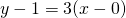# Solution assignment 10 Implicit differentiation

### Assignment 10

The equation:defines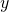as a differentiable function ofclose to the point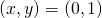.

Calculate the tangent line of the graph at this point.

### Solution

First we verify whether the point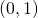lies on the graph of the expression, which appears to be the case.
Next we have to determine the derivative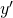and do this by implicit differentiation in combination with the product and quotient rule: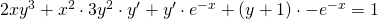For the pointwe get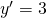The equation of the tangent line through the pointof the graph is: